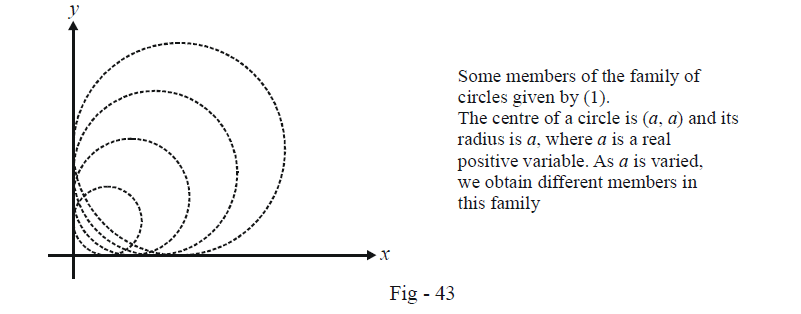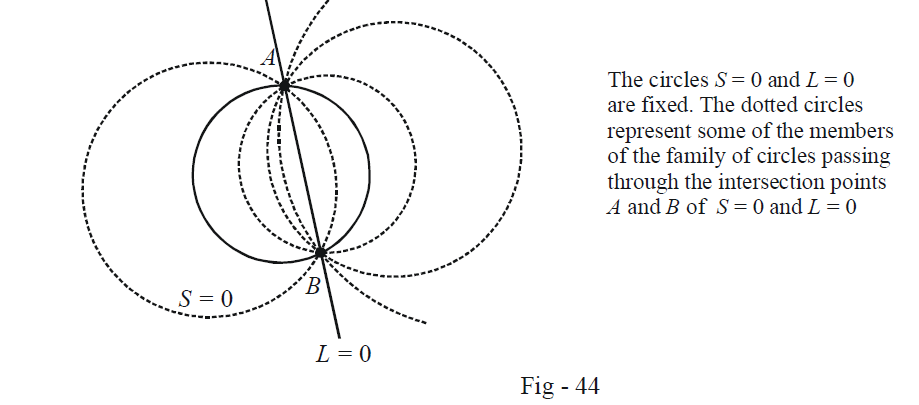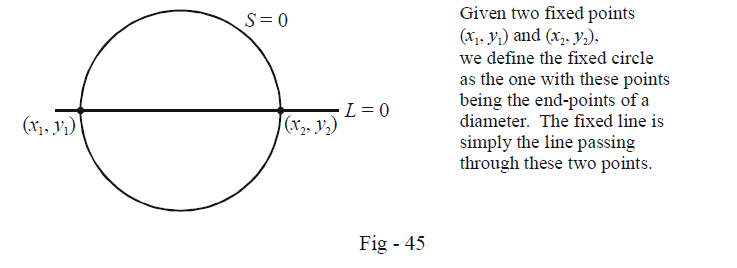# Family of Circles Type 1

Go back to  'Circles'

By a family of circles, we will mean a set of circles satisfying some given property (or properties). For example, the family of circles with each circle having its centre lying in the first quadrant and touching both the co-ordinate axes can be represented by the equation

${(x - a)^2} + {(y - a)^2} = {a^2}\,\,\,\,\,\,\,\,\,\,\,\,\,\,\,\,\,\,\,\,...(1)$

where a is a positive real number.

The important point to observe is that a is a variable here. As we vary a, we get different circles belonging to this family, but due to the constraint imposed by (1), all circles of this family satisfy the specified property.We intend to discuss in this section certain families that are of significant importance. In all cases, the family will be represented by an equation containing a real variable, which when varied will give rise to different members of this family.

TYPE 1:

\fbox{\begin{array}{*{20}{c}} \begin{align}&{\text{FAMILY OF CIRCLES PASSING THROUGH THE INTERSECTION POINTS OF }}\\&{\text{A GIVEN CIRCLE AND A GIVEN LINE}}\end{align}\end{array}}

We are given a fixed circle and a fixed line with equations $$S = 0$$ and $$L = 0$$ respectively. We want to find out the equation of the family of the circles passing through the points of intersection of $$S = 0$$ and $$L = 0$$ .By now, it should be apparent to you how to specify the equation of this family in terms of a real variable. Any circle F belonging to this family can be written as

\fbox{\begin{align} {F \equiv S + \lambda L = 0}\end{align}} where $$\lambda \in \mathbb{R}$$ .

The truth of this assertion can be easily verified. F as defined from this equation is definitely a circle since it satisfies the required constraints for its equation to be a circle. To see this, let

\begin{align}&S:{x^2} + {y^2} + 2gx + 2fy + c = 0\\&L:px + qy + r = 0\end{align}

We then have

\begin{align}&\,\,\,\,\,\,\,\,\,\,\,\,\,\,\,\,\,\,F:S + \lambda L = 0\\& \Rightarrow \quad\; {x^2} + {y^2} + (2g + \lambda p)x + (2f + \lambda q)y + c + \lambda r = 0\end{align}

which is definitely the equation of a circle. To see that F passes through A and B, note that since A and B satisfy both the equations $$S = 0$$ and $$L = 0,$$ they will obviously also satisfy the equation $$S + \lambda L = 0.$$

Thus, $$F \equiv S + \lambda L = 0$$ is the required family of circles. As we vary $$\lambda$$, we will get different circles belonging to this family. In particular, note that for $$\lambda = 0,$$ we get the circle $$S = 0$$ itself, while in the limit $$\lambda \to \infty ,$$ we get the ‘circle’ $$L = 0,$$ which is actually a line but can be considered a circle with centre lying at infinity and an infinitely large radius.

Example - 36

Write the equation for

(a)  the family of circles passing through $$({x_1},{y_1})$$ and $$({x_2},{y_2})$$

(b) the family of circles touching the line $$L = 0$$ at $$({x_1},{y_1})$$

Solution (a) Till now we have seen how to write the equation for a family of circles passing through the intersection points of a circle and a line. Thus, in the current example, we first define a fixed circle passing through $$({x_1},{y_1})$$ and $$({x_2},{y_2})$$ and the line through these two points.

The fixed circle S can easily be taken to be the one with $$({x_1},{y_1})$$ and$$({x_2},{y_2})$$ as the end points of a diameter:

$S:(x - {x_1})(x - {x_2}) + (y - {y_1})(y - {y_2}) = 0$

The fixed line can be written using the two-point form:

L:\frac{{y - {y_1}}}{{{y_2} - {y_1}}} = \frac{{x - {x_1}}}{{{x_2} - {x_1}}} \Rightarrow L:\left| {\begin{align}&x&&y&&1\\&{{x_1}}&&{{y_1}}&&1\\&{{x_2}}&&{{y_2}}&&1\end{align}} \right| = 0We can now write the required family of circles as:

$F:S + \lambda L = 0$

$\Rightarrow \ \fbox{(x - {x_1})(x - {x_2}) + (y - {y_2})(y - {y_2}) + \lambda L = 0}\qquad\qquad......(1)$

where $$L = 0$$ represents the line through the two given points

(b) We now want the family of circles to be such that each circle touches $$L = 0$$ at $$({x_1},{y_1})$$ .

We could evaluate it by letting $${x_2} \to {x_1}$$ and $${y_2} \to {y_1}$$ in (1). In this limit, $$L = 0$$  will simply become the tangent to any member of the family F given by (1). (Convince yourself about this point.)

Thus, the required family is

$\fbox{\begin{array}{*{20}{c}} {F':{{(x - {x_1})}^2} + {{(y - {y_1})}^2} + \lambda L = 0}\end{array}}$